CHAPTER 1

Chemical Reactions And Equations

Consider the following situations of daily life and think what happens when –

• milk  is  left  at  room  temperature  during  summers
• an  iron  tawa/pan/nail  is  left  exposed  to  humid  atmosphere
• grapes  get  fermented
• food is cooked
• food gets digested in our body
• we  respire

In  all  the  above  situations,  the  nature  and  the  identity  of  the  initial substance have somewhat changed. We have already learnt about physical and chemical changes of matter in our previous classes. Whenever a chemical change occurs, we can say that a chemical reaction has taken place.You may perhaps be wondering as to what is actually meant by a chemical reaction. How do we come to know that a chemical reaction has taken place? Let us perform some activities to find the answer to these questions.

Activity  1.1

CAUTION: This Activity needs the  teacher’s  assistance.  It Would  be  better  if  students wear  suitable  eye glasses

• Clean  a  magnesium  ribbon about 3-4 cm long by rubbing  with  sandpaper
• Hold  it  with  a  pair  of  tongs.Burn it using a spirit lamp orburner and collect the ash so formed  in  a  watch-glass  as shown  in  Fig.  1.1.  Burn    the magnesium ribbon keeping it away  as  far  as  possible  from your  eyes
• What  do  you  observe?Figure 1.1 Burning of a magnesium ribbon in air and collection of magnesium oxide in a watch-glass

You  must  have  observed  that  magnesium  ribbon  burns  with  dazzling white flame and changes into a white powder. This powder is magnesium oxide. It is formed due to the reaction between magnesium and oxygen present in the air.

Activity  1.2

• Take lead nitrate solution in a test tube
• Add  potassium iodide  solution to  this
• What  do  you observe?

Activity  1.3

• Take  a  few  zinc  granules  in  a  conical  flask  or  a  test  tube
• Add  dilute  hydrochloric  acid  or  sulphuric  acid  to  this(Fig. 1.2). CAUTION:  Handle  the  acid  with  care
• Do  you  observe  anything  happening  around  the  zinc granules
• Touch  the  conical  flask  or  test  tube.  Is  there  any  change  inits  temperature?Figure 1.2 Formation of hydrogen gas  by  the  action  of dilute sulphuric acid on zinc

From the above three activities, we can say that any of the following observations helps us to determine whether a chemical reaction has taken place –

• change in state
• change in colour
• evolution of a gas
• change in temperature.

As we observe the changes around us, we can see that there is a large variety of chemical reactions taking place around us. We will study about the various types of chemical reactions and their symbolic representation in this Chapter.

1.1 CHEMICAL EQUATIONS

Activity 1.1 can be described as — when a magnesium ribbon is burnt in oxygen, it gets converted to magnesium oxide. This description of a chemical reaction in a sentence form is quite long. It can be written in a shorter form. The simplest way to do this is to write it in the form of a word-equation.

The word-equation for the above reaction would be —

Magnesium  +  Oxygen    —r Magnesium oxide   (l.l)

(Reactants)                              (Product)

The substances that undergo chemical change in the reaction (1.1), magnesium and oxygen, are the reactants. The new substance is magnesium oxide, formed during the reaction, as a product.

A word-equation shows change of reactants to products through an arrow placed between them. The reactants are written on the left-hand side (LHS) with a plus sign (+) between them. Similarly, products are written on the right-hand side (RHS) with a plus sign (+) between them. The arrowhead points towards the products, and shows the direction of the reaction.

1.1.1 Writing a Chemical Equation

Is there any other shorter way for representing chemical equations? Chemical equations can be made more concise and useful if we use chemical formulae instead of words. A chemical equation represents a chemical reaction. If you recall formulae of magnesium, oxygen and magnesium oxide, the above word-equation can be written as —Count and compare the number of atoms of each element on the LHS and RHS of the arrow. Is the number of atoms of each element the same on both the sides? If yes, then the equation is balanced.  If  not, then the equation is unbalanced because the mass is not the same  on both sides of the equation. Such a chemical equation is a skeletal chemical equation for a reaction. Equation (1.2) is a skeletal chemical equation for the burning of magnesium in air.

### 1.1.9 Balanced Chemical Equations

Recall the law of conservation of mass that you studied in Class IX; mass can neither be created nor destroyed in a chemical reaction. That is, the total mass of the elements present in the products of a chemical reaction has to be equal to the total mass of the elements present in the reactants. In other words, the number of atoms of each element remains the same, before and after a chemical reaction. Hence, we need to balance a skeletal chemical equation. Is the chemical Eq. (1.2) balanced? Let us

learn about balancing a chemical equation step by step.

The word-equation for Activity 1.3 may be represented as —

Zinc + Sulphuric acid —r Zinc sulphate + Hydrogen

The above word-equation may be represented by the following chemical equation —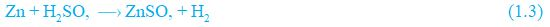Let us examine the number of atoms of different elements on both sides of the arrow.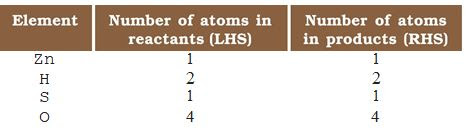As the number of atoms of each element is the same on both sides of the arrow, Eq. (1.3) is a balanced chemical equation.

Let us try to balance the following chemical equation —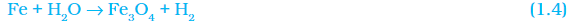Step I: To balance a chemical equation, first draw boxes around each formula. Do not change anything inside the boxes while balancing the equation.Step II: List the number of atoms of different elements present in the Unbalanced equation (1.5).Step III: It is often convenient to start balancing with the compound that contains the maximum number of atoms. It may be a reactant or a product. In that compound, select the element which has the maximum number of atoms. Using these criteria, we select Fe,O4 and the element

oxygen in it. There are four oxygen atoms on the RHS and   only one on the LHS.

To balance the oxygen atoms —To equalize the number of atoms, it must be remembered that we cannot alter the formulae of the compounds or elements involved in the reactions. For example, to balance oxygen atoms we can put coefficient

‘4’ as 4 H2O and not H2O4 or (H2O)4 . Now the partly balanced equation becomes —

Fe + 4 H2O Fe3O4 + H2  (partly balanced equation) (1.6)

Step IV: Fe and H atoms are still not balanced. Pick any of these elements to proceed further. Let us balance hydrogen atoms in the partly balanced equation.

To equalise the number of H atoms, matte the number of molecules of hydrogen as four on the RHS.The equation would be —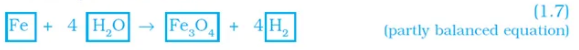Step V: Examine the above equation and pick up the third element which is not balanced. You find that only one element is left to be balanced, that is, iron.To equalise Fe, we take three atoms of  Fe on  the  LHS.Step VI: Finally, to check the correctness of the balanced equation, we count atoms of each element on both sides of the equation.The numbers of atoms of elements on both sides of Eq. (1.9) are equal. This equation is now balanced. This method of balancing chemical equations is called hit-and-trial method as we make trials to balance the equation by using the smallest whole number coefficient.

Step VII: Writing Symbols OJ Physical Stotes Carefully examine the above balanced Eq. (1.9). Does this equation tell us anything about the physical state of each reactant and product? No information has been given in this equation about their physical states.

To make a chemical equation more informative, the  physical states of the reactants and products are mentioned along with their chemical formulae. The gaseous, liquid, aqueous and solid  states  of  reactants and products are represented by the notations (g), (l), (aq) and (s), respectively. The word aqueous (aq) is written if the reactant or product is present as a solution in water.

The balanced Eq. (1.9) becomesNote that the symbol (g) is used with H2O to indicate that in this reaction water is used in the form of steam.

Usually physical states are not included in a chemical equation urdess it is necessary to specify them.

Sometimes the reaction conditions, such as temperature, pressure, catalyst, etc., for the reaction are indicated above and/or below the arrow in the equation. For example —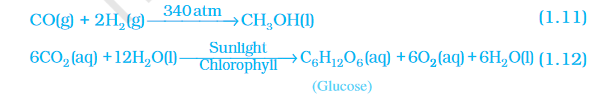Using these steps, can you balance Eq. (1.2) given in the text earlier?

# Questions

Q1 Why should a magnesium ribbon be cleaned before burning in air?

Ans-  The magnesium ribbon should be cleaned before burning in the air because the layer of magnesium oxide (which is formed due to the reaction of magnesium with air ) can be removed in order to get the desired chemical reaction.
Also in order to remove the oxide layer, the presence of which slowdowns the oxidation process. So, by cleaning it, the burning process takes place easily.

Q2. Write the balanced equation for the following chemical reactions.

(i)Hydrogen + Chlorine Hydrogen chloride

H2 + Cl2 → HCl
H2 + Cl2 → 2HCl

(ii) Barium chloride + Aluminium sulphate Barium sulphate + Aluminium chloride

BaCl2 + Al2(SO4)3 → BaSO4 + AlCl3
3BaCl2 + (Al2  SO4)3 →3BaSO4 + 2AlCl3

(iii) Sodium + Water Sodium hydroxide + Hydrogen
Na + H2O →  NaOH + H2
2Na + 2H2O → 2NaOH + H2

Q3. Write a balanced chemical equation with state symbols for thefollowing reactions.

(a) Solutions of barium chloride and sodium sulphate in water react to give insoluble barium sulphate and the solution of sodium chloride.

Ans:  BaCl2 (aq) + Na2SO4 ? BaSO4 (s) + 2NaCl (aq)

(b) Sodium hydroxide solution (in water) reacts with hydrochloric acid solution (in water) to produce sodium chloride solution and water.

Ans :  NaOH (aq) + HCl ? NaCl (aq) + H2O (l)

1.2 TYPES OF CHEMICAL REACTIONS

We have learnt in Class IX that during a chemical reaction atoms of one element do not change into those of another element. Nor do atoms disappear from the mixture or appear from elsewhere. Actually, chemical reactions involve the breaking and making of bonds between atoms to produce new substances. You will study about types of bonds formed between atoms in Chapters 3 and 4.

1.2.1 Combination Reaction

Activity  1.4

• Take  a  small  amount  of  calcium  oxideor quick lime in a beaker
• Slowly  add  water  to  this
• Touch the beaker as shown in Fig. 1.3
• Do you feel any change in temperature?Figure 1.3 Formation  of  slaked lime by the reaction of calcium  oxide  with water

Calcium oxide reacts vigorously with water to produce slaked lime (calcium hydroxide) releasing a large amount of heat.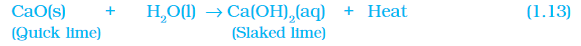In this reaction, calcium oxide and water combine to form a single product, calcium hydroxide. Such a reaction in which a single product isformed from two or more reactants is known as a combination reaction.

Do You Know?

A solution of slaked lime produced by the reaction 1. l3 is used for whitewashing walls. Calcium hydroxide reacts slowly with the carbon dioxide in air to form a thin

layer of calcium carbonate on the walls. Calcium carbonate is formed after two to  three days of whitewashing and gives a shiny rinish to the walls. It is interesting to note that the chemical formula for marble is also CaCO3.

Ca(OH)2(aq) + CO2(g)CaCO3(s) + H2O(l)    (1.14)

(Calcium hydroxide)   (Calcium carbonate)

Let us discuss some more examples of combination reactions.

1.  Burning of coal1. Formation of water from H2(g) and O2(g)In simple language we can say that when two or more substances (elements or compounds) combine to form a single product, the reactions are called combination reactions.

In Activity 1.4, we also observed that a large amount of heat is evolved. This makes the reaction mixture warm. Reactions in which heat is released along with the formation of products are called exothermic chemical reactions.

Other examples of exothermic reactions are —

1. Burning of natural gas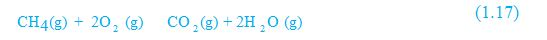1. Do you know that respiration is an exothermic process?

We all know that we need energy to stay alive. We get this energy from the food we eat. During digestion, food is brohen down into simpler substances. For example, rice, potatoes and bread contain carbohydrates. These carbohydrates are broken down to form glucose. This glucose combines with oxygen in the cells of our body and provides energy. The special name of this reaction is respiration, the process of which you will study in Chapter 6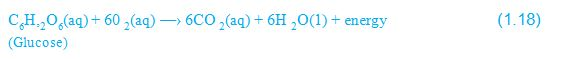3. The decomposition of vegetable matter into compost is also an example of an exothermic reaction.

Identify the type of the reaction taking place in Activity 1. 1, where heat is given out along with the formation of a single product.